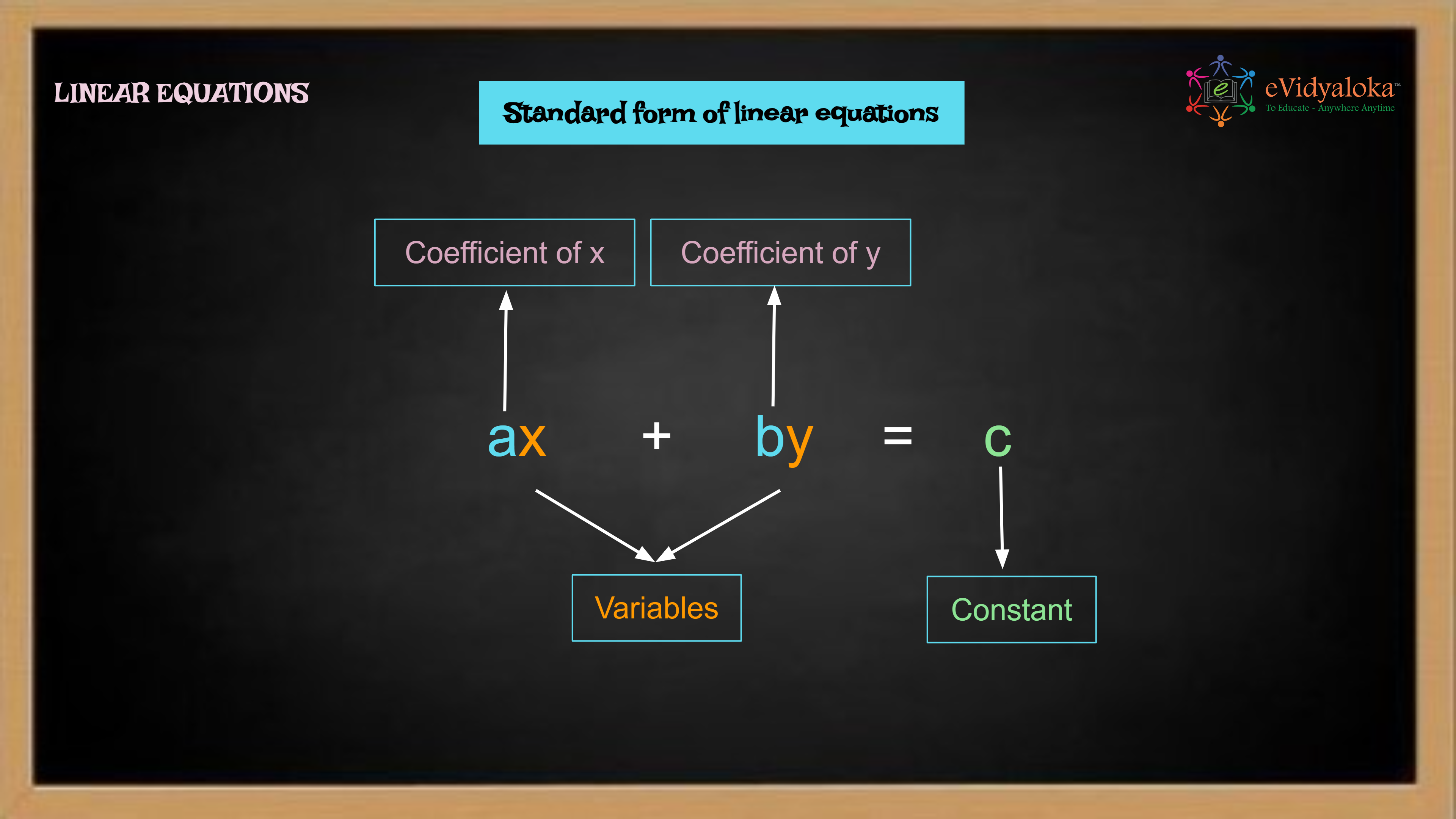# Application of Linear Equations

``````

Dear Volunteer Teacher,

Kindly teach the bridge concept of Application of Linear Equations prior to teaching the related textbook chapters.
Thank you.

## Course Content

• Linear and non-linear equations
• Standard form of linear equation
• Linear equation with one variable
• Solving linear equation with one variable
• Reducing an equation to linear form
• Application of linear equation

## Learning Outcomes

Learning outcomes has been listed below which will serve as your guide for what students need to learn.

SWBAT:

• define a Linear equation and represent it its standard form and with variable on only one side and also equations with variables on both sides.
• solve the above type of linear equations with one variable.
• reduce equation to linear form based on cross multiuplication.

## PPT Deck

To make the lesson come alive, please find below PPT deck